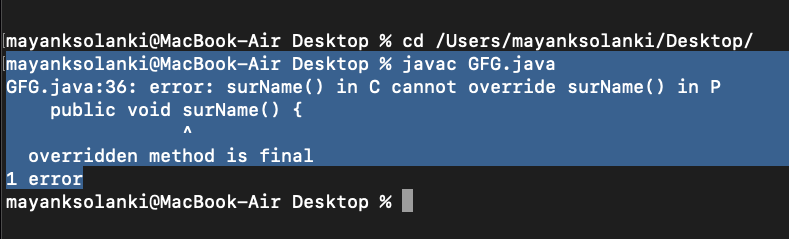Open in App
Not now

# Java – Final vs Static Access Modifier

• Last Updated : 05 Jan, 2023

Final keyword is used in different contexts. First of all, final is a non-access modifier applicable only to a variable, a method, or a class. Following are different contexts where final is used. While the static keyword in Java is mainly used for memory management. The static keyword in Java is used to share the same variable or method of a given class. The users can apply static keywords with variables, methods, blocks, and nested classes.

### Final Access Modifier

Final access modifier is a modifier applicable to classes, methods, and variables. If we declare a parent class method as final then we can’t override that method in the child class because its implementation is final and if a class is declared as final we can’t extend the functionality of that class i.e we can’t create a child class for that class i.e inheritance is not possible for final classes. Every method present inside the final class is always final y default but every variable present inside the final class need not be final. The main advantage of the final keyword is we can achieve security and we can provide a unique implementation. But the main disadvantage of the final keyword is we are missing key benefits of OOPs like Inheritance(Because of the final class), Polymorphism(Because of the final method)  hence if there are no specific requirements then it is not recommended to use the final keyword.

Example 1:

## Java

 `// Java Program to illustrate Final keyword` `// Where No final keyword Is Used`   `// Importing required classes` `import` `java.io.*;` `import` `java.util.*;`   `// Class 1` `// Super-class` `class` `P {`   `    ``// Method 1` `    ``// To declare first name` `    ``public` `void` `firstName()` `    ``{`   `        ``// Passing name and print it` `        ``System.out.println(``"Mayank"``);` `    ``}`   `    ``// Method 2` `    ``// To declare last name` `    ``public` `void` `surName()` `    ``{`   `        ``// Passing name and print it` `        ``System.out.println(``"Trivedi"``);` `    ``}` `}`   `// Class 2` `// Sub-class` `// Extending above class` `class` `C ``extends` `P {`   `    ``// Method 1` `    ``// Trying to override the last name` `    ``public` `void` `surName()` `    ``{` `        ``// Display surname` `        ``System.out.println(``"Sharma"``);` `    ``}`   `    ``// Method 2` `    ``// Main driver method` `    ``public` `static` `void` `main(String[] args)` `    ``{`   `        ``// Display message` `        ``System.out.println(``"GFG"``);` `    ``}` `}`

Output

`GFG`

Example 2:

## Java

 `// Java Program to illustrate Final keyword` `// When final keyword Is Used`   `// Importing required classes` `import` `java.io.*;` `import` `java.util.*;`   `// Class 1` `// Super-class` `class` `P {`   `    ``// Method 1` `    ``// To declare first name` `    ``public` `void` `firstName()` `    ``{`   `        ``// Passing name and print it` `        ``System.out.println(``"Mayank"``);` `    ``}`   `    ``// Method 2` `    ``// To declare last name` `    ``public` `final` `void` `surName()` `    ``{`   `        ``// Passing name and print it` `        ``System.out.println(``"Trivedi"``);` `    ``}` `}`   `// Class 2` `// Sub-class` `// Extending above class` `class` `C ``extends` `P {`   `    ``// Method 1` `    ``// Trying to override the last name` `    ``public` `void` `surName()` `    ``{` `        ``// Display surname` `        ``System.out.println(``"Sharma"``);` `    ``}`   `    ``// Method 2` `    ``// Main driver method` `    ``public` `static` `void` `main(String[] args)` `    ``{`   `        ``// Display message` `        ``System.out.println(``"GFG"``);` `    ``}` `}`

Output:### Static Access Modifier

Static access modifier is an access modifier that is applicable for methods and variables but not for classes. We can declare top-level class with a static modifier but we can declare the inner class as static (such types of inner classes are known as static nested classes). In the case of instance variable for every object, a separate copy will be created but in the case of static variable, a single copy will be created at class level and shared by every object of that class.

Example

## Java

 `// Java Program to Illustrate Static Access Modifier`   `// Importing required classes` `import` `java.io.*;` `import` `java.util.*;`   `// Main class` `class` `GFG {`   `    ``// Creating a static variable and` `    ``// initializing a custom value` `    ``static` `int` `x = ``10``;`   `    ``// Creating a instance variable and` `    ``// initializing a custom value` `    ``int` `y = ``20``;`   `    ``// Main driver method` `    ``public` `static` `void` `main(String[] args)` `    ``{`   `        ``// Creating an object of class inside main() method` `        ``GFG t1 = ``new` `GFG();`   `        ``// Accessing and re-initializing the` `        ``// static and instance variable` `        ``// using t1 reference` `        ``t1.x = ``88``;` `        ``t1.y = ``99``;`   `        ``// Creating an object of class inside main() method` `        ``// again` `        ``GFG t2 = ``new` `GFG();`   `        ``// Accessing the static and instance variable using` `        ``// t2 reference as we know that for each object` `        ``// there is a separate copy of instance variable` `        ``// created. While a same copy of static variable will` `        ``// be shared between the objects`   `        ``// Displaying the value of static and instance` `        ``// variable using t2 object reference` `        ``System.out.println(` `            ``"Value of Static variable x = "` `+ t2.x + ``"\n"` `            ``+ ``"Value of Instance variable y = "` `+ t2.y);` `    ``}` `}`

Output

```Value of Static variable x = 88
Value of Instance variable y = 20```

After having a good understanding and implementation of both of the above specifiers and these important special keywords let us tabulate differences between them to grasp a good understanding over these keywords.

My Personal Notes arrow_drop_up
Related Articles